So far we have worked with the most essential concepts of Python: variables, data structures, built-in functions and methods, for loops, and if statements. These are all parts of the core semantics of the language. But this is far from everything that Python knows… actually this is just the very beginning and the exciting stuff is yet to come. Because Python also has tons of modules and packages that we can import into our projects… What does this mean? In this article I’ll give you an intro: I’ll show you the Python `import` statement and the most important built-in modules that you have to know for data science!

## Before we start

If you haven’t done so yet, I recommend going through these articles first:

## The Python Import Statement

Okay, so what is the `import` statement and why is it so important?

Think of it like LEGO:Python core semantics

So far we have played around with the base elements of our LEGO playset. But if you want to build something complex, you have to use more advanced tools.

If you use `import`, you can get access to the advanced Python “tools” (they are called modules).New tools to access via the Python import statement

These are divided into three groups:

1. The modules of the Python Standard Library:
You can get these really easily because they come with Python3 by default. You simply have to type `import` and the name of the module – and from that point on you can use the given module in your code. In this article, I’ll show you exactly how to do that in detail.
2. Other, even more advanced and more specialized modules:
There are modules that are not part of the standard library. For these, you have to install new packages to your data server first. You will see that for data science we are using many of these “external” packages. (The ones you might have heard about are pandas, numpy, matplotlib, scikit-learn, etc.) I’ll get back to this topic in another article.
Yes, you can write new modules by yourself, too! (I’ll cover this in my advanced Python tutorials.)

Anyway, `import` is a really powerful concept in Python – because with that you’ll be able to expand your toolset continuously and almost infinitely when you are dealing with different data science challenges.

## The most important Python Built-in Modules for Data Scientists

Okay, now that you get the concept, it’s time to see it in practice. As I have mentioned, there is a Python Standard Library with dozens of built-in modules. From those, I hand-picked the five most important modules for data analysts and scientists. These are:

• random
• statistics
• math
• datetime
• csv

You can easily `import` any of them by using this syntax:

`import [module_name]`

eg. `import random`

Note: This will import the entire module with all items in it. You can import only a part of the module, too: `from [module_name] import [item_name]`. But let’s not complicate things with that yet.

Let’s see the five built-in modules one by one!

## Python Built-in Module #1: random

Randomization is very important in data science… just think about experimenting and A/B testing! If you import the random module, you can generate random numbers by various rules.

Let’s type this to your Jupyter Notebook first:
`import random`

Then in a separate cell try out:
`random.random()`

This will generate a random float between 0 and 1.

Try this one, too:
`random.randint(1,10)`

This will generate a random integer between 1 and 10.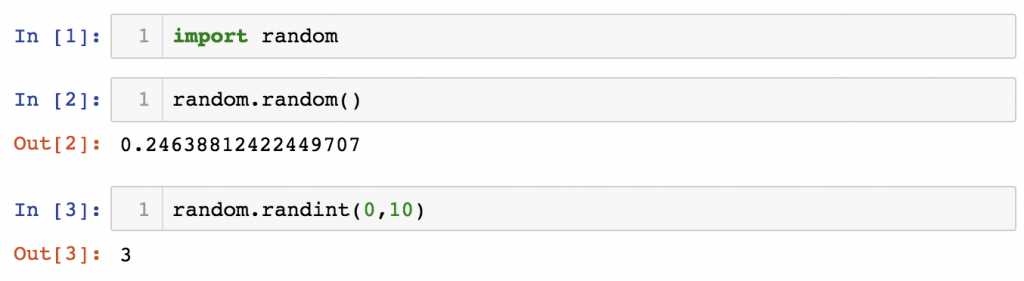Learn more about `random`here.

## Python Built-in Module #2: statistics

If randomization is important, statistics is inevitable!
Lucky for us, there is a statistics built-in module which contains functions like: mean, median, mode, standard deviation, variance and more…

Let’s try few of these:
`import statistics`

Create a sample list:
`a = [0, 1, 1, 3, 4, 9, 15]`

Then calculate all the above mentioned values for this small list:

```statistics.mean(a)
statistics.median(a)
statistics.mode(a)
statistics.stdev(a)
statistics.variance(a)
```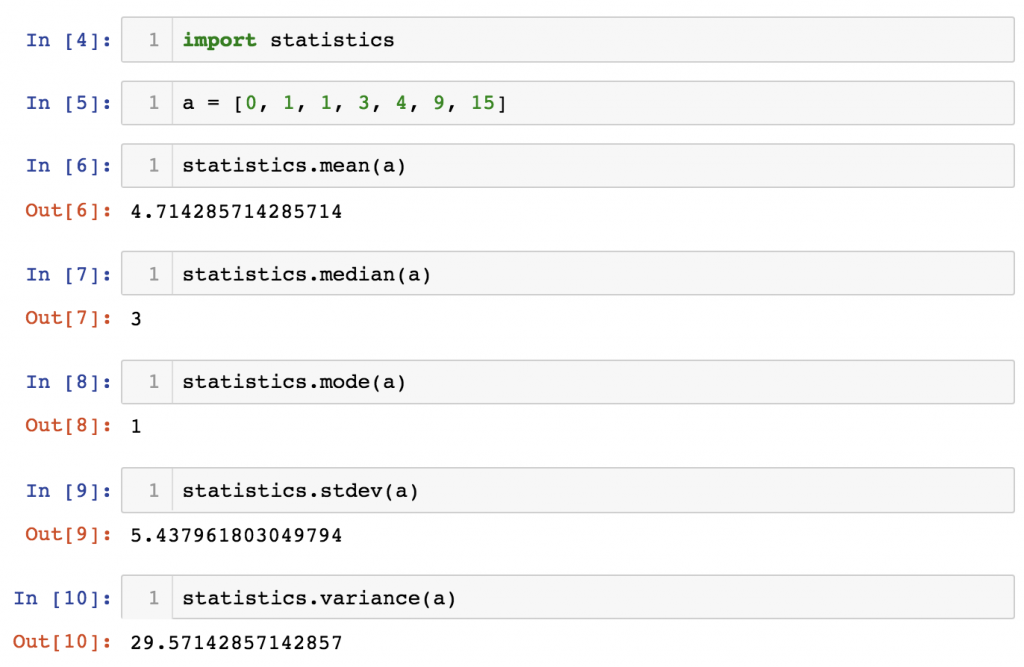Learn more about the `statistics` module here.

## Python Built-in Module #3: math

There are a few functions that are under the umbrella of math rather than statistics. So there is a separate module for that. This contains factorial, power, and logarithmic functions, but also some trigonometry and constants.

Try this:
`import math`

And then:

```math.factorial(5)
math.pi
math.sqrt(5)
math.log(256, 2)
```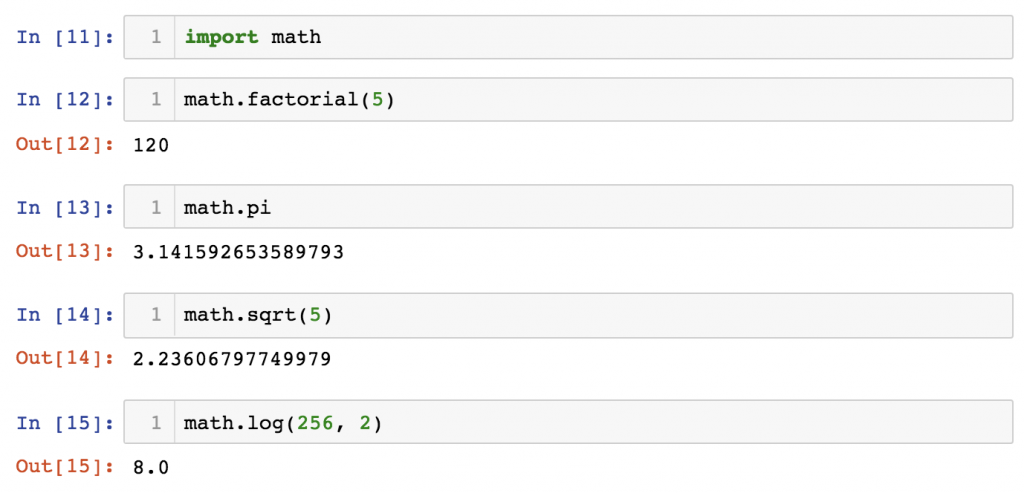Learn more about `math` module here.

## Python Built-in Module #4: datetime

Do you plan to work for an online startup? Then you will probably encounter lot of data logs. And the heart of a data log is the datetime. Python3, by default, does not handle dates and times, but if you import the datetime module, you will get access to these functions, too.

`import datetime`

To be honest, I think the implementation of the datetime module of Python is a bit over-complicated… at least, it’s not easy to use for beginners. I’ll write a separate article about it later. But for now let’s try these two functions to get a bit more familiar with it:

`datetime.datetime.now()`
`datetime.datetime.now().strftime("%F")`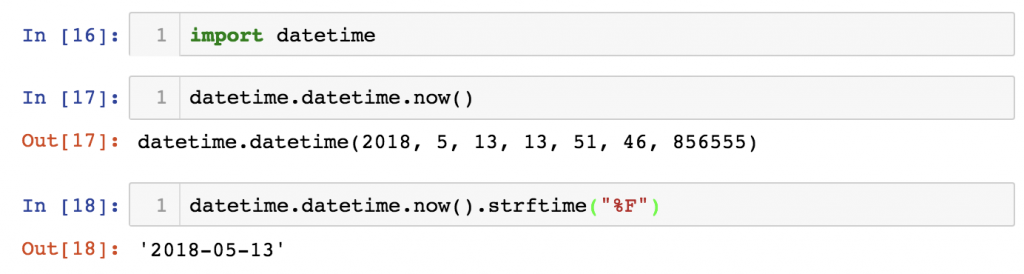## Python Built-in Module #5: csv

“csv” stands for “comma-separated values” and it’s one of the most common file formats for plain text data logs. So you definitely have to know how to open a .csv file in Python. There is a certain way to do that – just follow this example.

Let’s say you have this small .csv file. (You can even create it in Jupyter.) fruits.csv:

```2018-04-10;1001;banana
2018-04-11;1002;orange
2018-04-12;1003;apple
```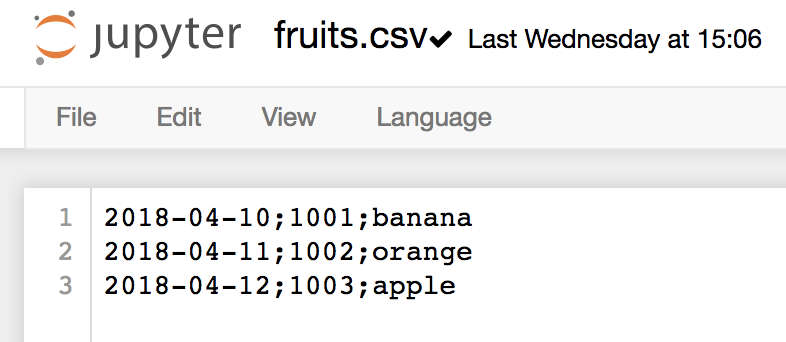If you want to open this file in your Jupyter Notebook, you have to apply this code:

```import csv

with open('fruits.csv') as csvfile:
for row in my_csv_file:
print(row)
```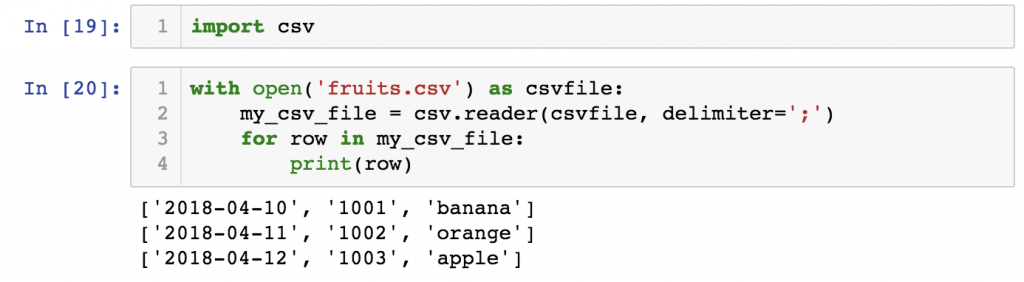As you can see, it returned Python lists. So with the list selection features and with the list methods that we have learned previously, you can also break down and restructure this data.

## More built-in modules

This is a good start but far from the whole list of the Python built-in modules. With other modules you can zip and unzip files, scrape websites, send emails, encode and decode JSON files and do a lot of other exciting things. If you want to take a look at the whole list, check out the Python Standard Library which is part of the original Python documentation.
And, as I mentioned, there are other Python libraries and packages that are not part of the standard library (like pandas, numpy, scipy, etc.) – I’ll write more about them soon!

## Syntax

Now that you have seen how `import` works, let’s talk briefly about the syntax!

Three things:

1. Usually, in Python scripts, we put all the `import` statements at the beginning of our script. Why is that? To see what modules our script relies on. Also, to make sure that the modules will be imported before we need to apply them. So keep this advice in mind: `import` statements come at the beginning of your Python scripts.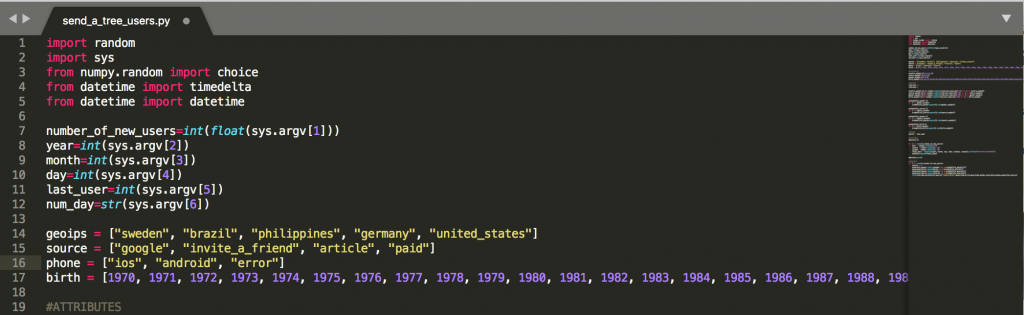2. In this article, we applied the functions of the modules using this syntax:
`module_name.function_name(parameters)`
Eg. `statistics.median(a)`
or
`csv.reader(csvfile, delimiter=';')`This is logical: before you apply a given function, you have to tell Python in which module to find it.In some cases there are even more complicated relationships – like functions of classes in a module (eg. `datetime.datetime.now())` but let’s not confuse yourself with that for now. My suggestion is to make a list of your favorite modules and functions and learn how they work; if you need a new one, check out the original Python documentation and add the new module plus its function to your list.
3. When you import a module (or a package) you can rename it using the `as` keyword:
If you type:
`import statistics as stat`
you have to refer to your module as stat. Eg. `stat.median(a)` and not as `statistics.median(a)`.Conventionally, we are using two very well-known data science related Python libraries imported with their shortened name: numpy (`import numpy as np`) and pandas (`import pandas as pd`). I’ll get back to this in another article!

## So what’s the name of it? Package? Module? Function? Library?

When I first encountered this `import` concept, I had a hard time understanding what exactly I was importing. In some cases these things were referred as “modules”, some cases as “packages”, in other cases as “functions”, and sometimes as “libraries”.

Note: Even if you check numpy and pandas – the two most popular data science related python libraries (or packages??). One is called library, the other is called a package.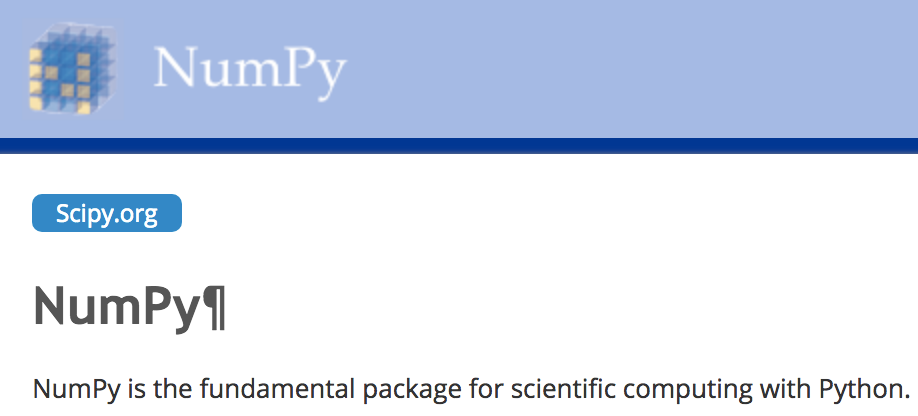the numpy >>package<<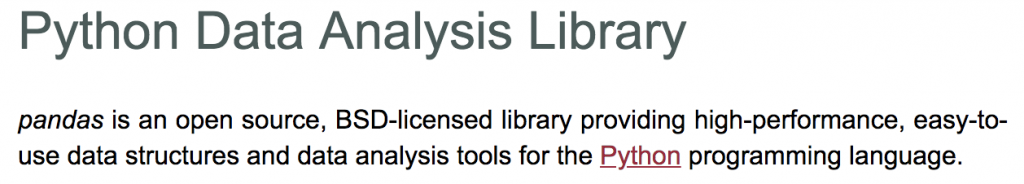the pandas >>library<<

Truth be told, I’m not a big fan of academical or theoretical questions in programming, like how we name things. But I have to admit that if you want to keep up a meaningful discussion with a developer (or even just ask a question on Stackoverflow), you have to have at least a clue about what is what.

So here is the result of my research – a little bit simplified of course:

• Function: it’s a block of code that you can (re-)use by calling it with a keyword. Eg. `print()` is a function.
• Module: it’s a .py file that contains a list of functions (it can also contain variables and classes). Eg. in `statistics.mean(a)`, `mean` is a function that is found in the `statistics` module.
• Package: it’s a collection of Python modules. Eg. `numpy.random.randint(2, size=10)` `randint()` is a function in the `random` module of the `numpy` package.
• Library: it’s a more general term for a collection of Python codes.

The best answers I found on this topic are below this Stackoverflow question.

## Conclusion

`import` is an essential and powerful concept within Python. The more you learn about data science, the better you will understand that you will have to continuously expand your toolset for the different challenges you will face. `import` is the ultimate tool for that. It opens up thousands of new doors. In my upcoming articles I’ll introduce the most important Python libraries and packages you have to know as a data scientist!

Cheers,
Tomi Mester